# Hypercomplex number

(diff) ← Older revision | Latest revision (diff) | Newer revision → (diff)

An element of a finite-dimensional algebra with a unit element over the field of real numbers(formerly known as a hypercomplex system). Historically, hypercomplex numbers arose as a generalization of complex numbers (cf. Complex number). Operations on complex numbers correspond to geometrical transformations of the plane (translation, rotation, dilation, and combinations of such operations). In trying to construct numbers whose role with respect to three-dimensional space corresponds to the role played by complex numbers with respect to the plane, it became clear that a full analogy is not possible; this gave rise to the development of the theory of systems of hypercomplex numbers.

A hypercomplex system of rankis obtained by introducing a multiplication in the-dimensional real spacewhich satisfies the axioms of an algebra over a field. Let 1 be the unit of a hypercomplex systemand let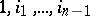be some basis of. The hypercomplex number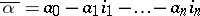ofis said to be the conjugate hypercomplex number of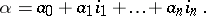Let, where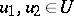andis some new symbol. The set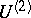may be converted into a hypercomplex system by defining addition byand multiplication byThe hypercomplex systemis called the doubling of.

Examples of hypercomplex systems are: the real numbers, the complex numbers, the quaternions, and the Cayley numbers (in this list each successive system is obtained by doubling the preceding one, cf. Quaternion; Cayley numbers). Other examples include double and dual numbers, and hypercomplex systems of the form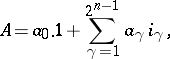which, if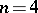, are known as Clifford–Lipschitz numbers (these hypercomplex numbers are elements of the Clifford algebra of rank). An important example of hypercomplex systems are complete matrix algebras over.

The definition of a system of hypercomplex numbers may include the requirement of associativeness of multiplication; one also identifies the concepts of an algebra and a hypercomplex system.

How to Cite This Entry:
Hypercomplex number. Encyclopedia of Mathematics. URL: http://encyclopediaofmath.org/index.php?title=Hypercomplex_number&oldid=18770
This article was adapted from an original article by N.N. Vil'yams (originator), which appeared in Encyclopedia of Mathematics - ISBN 1402006098. See original article